# Clustering Validation¶

We will play with data blobs with different characteristics

In :
from pylab import *
import matplotlib.pyplot as plt
from sklearn.decomposition import PCA
from mpl_toolkits.mplot3d import Axes3D
from amltlearn.datasets import make_blobs
from sklearn.cluster import KMeans
from sklearn.mixture import GaussianMixture
%matplotlib notebook

blobs, blabels = make_blobs(n_samples=200, n_features=3, centers=[[1,1,1],[0,0,0],[-1,-1,-1]], cluster_std=[0.2,0.1,0.3])

fig = plt.figure(figsize=(10,10))

ax = fig.add_subplot(111, projection='3d')
plt.scatter(blobs[:, 0], blobs[:, 1], zs=blobs[:, 2], depthshade=False, c=blabels, s=100)
plt.show()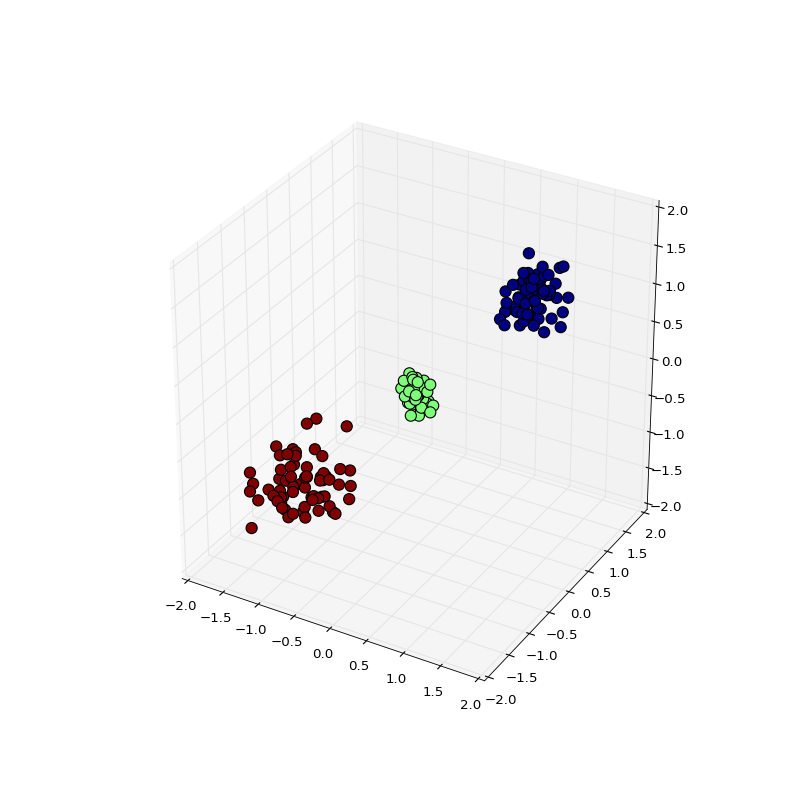These are very well separated blobs very easy to discover using k-means

In :
km = KMeans(n_clusters=3, n_init=10, random_state=0)
labels = km.fit_predict(blobs)

In :
from amltlearn.metrics.cluster import calinski_harabasz_score, davies_bouldin_score
from sklearn.metrics import adjusted_mutual_info_score, silhouette_score

lscores = []
nclusters = 5
for nc in range(2,nclusters+1):
km = KMeans(n_clusters=nc, n_init=10, random_state=0)
labels = km.fit_predict(blobs)
lscores.append((
silhouette_score(blobs, labels),
calinski_harabasz_score(blobs, labels),
davies_bouldin_score(blobs, labels)))

fig = plt.figure(figsize=(15,5))
plt.plot(range(2,nclusters+1), [x for x,_,_ in lscores])
plt.plot(range(2,nclusters+1), [x for _, x,_ in lscores])
plt.plot(range(2,nclusters+1), [x for _, _, x in lscores])

plt.show()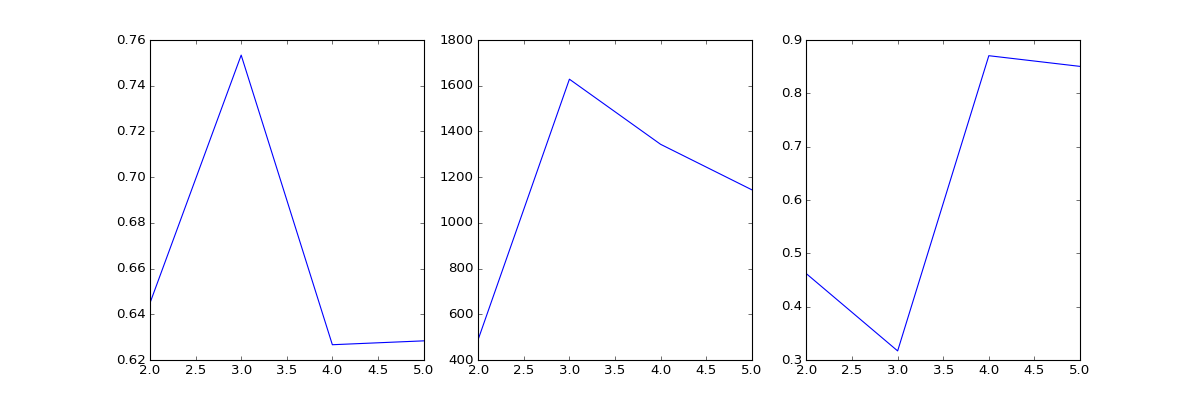The Silhouette (higher), CH (higher) and DB (lower) indices aggree on that there are three clusters on the data

Let's introduce some overlapping among the clusters

In :
blobs, blabels = make_blobs(n_samples=200, n_features=3, centers=[[1,1,1],[0,0,0],[-1,-1,-1]], cluster_std=[0.4,0.45,0.3])

fig = plt.figure(figsize=(10,10))

ax = fig.add_subplot(111, projection='3d')
ax.view_init(60, 90)
plt.scatter(blobs[:, 0], blobs[:, 1], zs=blobs[:, 2], depthshade=False, c=blabels, s=100)
plt.show()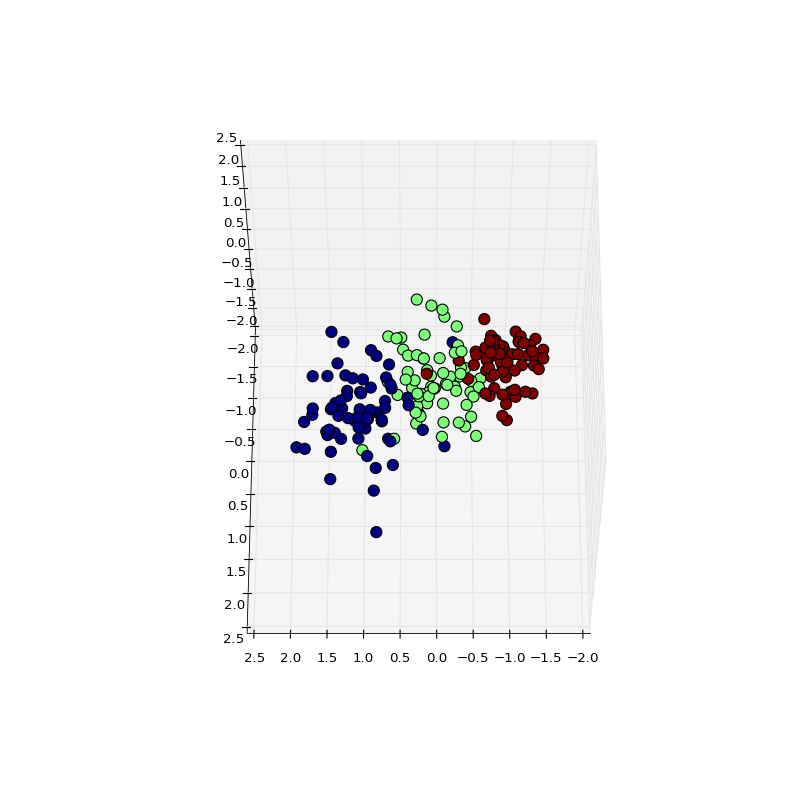Now is a little bit harder for k-means to discover the clusters

In :
km = KMeans(n_clusters=3, n_init=10, random_state=0)
labels = km.fit_predict(blobs)


0.87834389552

In :
lscores = []
nclusters = 5
for nc in range(2,nclusters+1):
km = KMeans(n_clusters=nc, n_init=10, random_state=0)
labels = km.fit_predict(blobs)
lscores.append((
silhouette_score(blobs, labels),
calinski_harabasz_score(blobs, labels),
davies_bouldin_score(blobs, labels)))

fig = plt.figure(figsize=(15,5))
plt.plot(range(2,nclusters+1), [x for x,_,_ in lscores])
plt.plot(range(2,nclusters+1), [x for _, x,_ in lscores])
plt.plot(range(2,nclusters+1), [x for _, _, x in lscores])

plt.show()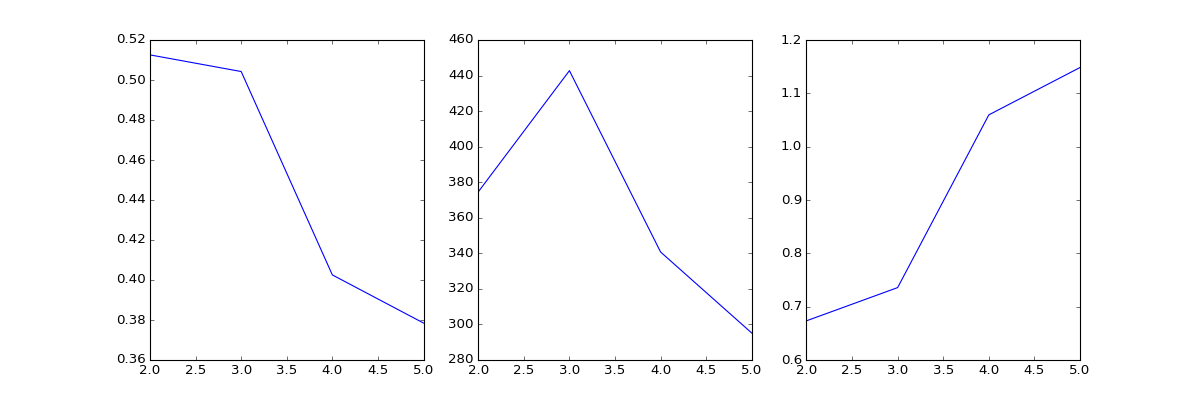Now only CH indicex says that there is three clusters on the dataset, Silhouette and DB only see two.

In :
from amltlearn.metrics import  scatter_matrices_scores

lscores = []
nclusters = 5
for nc in range(2,nclusters+1):
km = KMeans(n_clusters=nc, n_init=10, random_state=0)
labels = km.fit_predict(blobs)
lscores.append(scatter_matrices_scores(blobs, labels, indices= ['Hartigan', 'Xu', 'ZCF']))

fig = plt.figure(figsize=(15,5))
plt.plot(range(2,nclusters+1), [x['Hartigan'] for x in lscores])
plt.plot(range(2,nclusters+1), [x['ZCF'] for x in lscores])
plt.plot(range(2,nclusters+1), [x['Xu'] for x in lscores])

plt.show()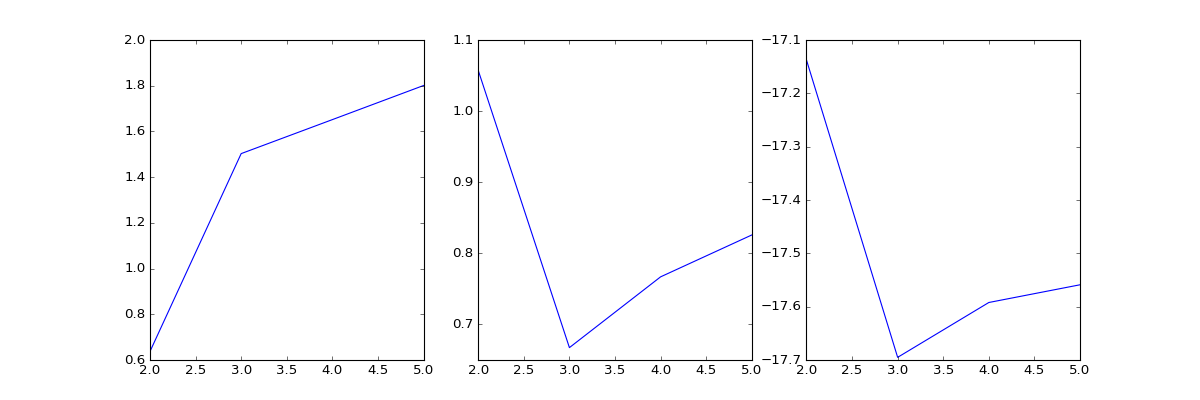These are the classical Hartigan index (used fequently for hierarchical clustering) and two other recent published indices

GMM does not have more luck discovering the noisy clusters.

In :
gmm = GaussianMixture(n_components=3, covariance_type='diag', random_state=0)
gmm.fit(blobs)

0.612492363836

In :
lscores = []
nclusters = 5
for nc in range(2,nclusters+1):
gmm = GaussianMixture(n_components=nc, covariance_type='diag', random_state=0)
gmm.fit(blobs)
labels = gmm.predict(blobs)
lscores.append((
silhouette_score(blobs, labels),
calinski_harabasz_score(blobs, labels),
davies_bouldin_score(blobs, labels)))

fig = plt.figure(figsize=(15,5))
plt.plot(range(2,nclusters+1), [x for x,_,_ in lscores])
plt.plot(range(2,nclusters+1), [x for _, x,_ in lscores])
plt.plot(range(2,nclusters+1), [x for _, _, x in lscores])

plt.show()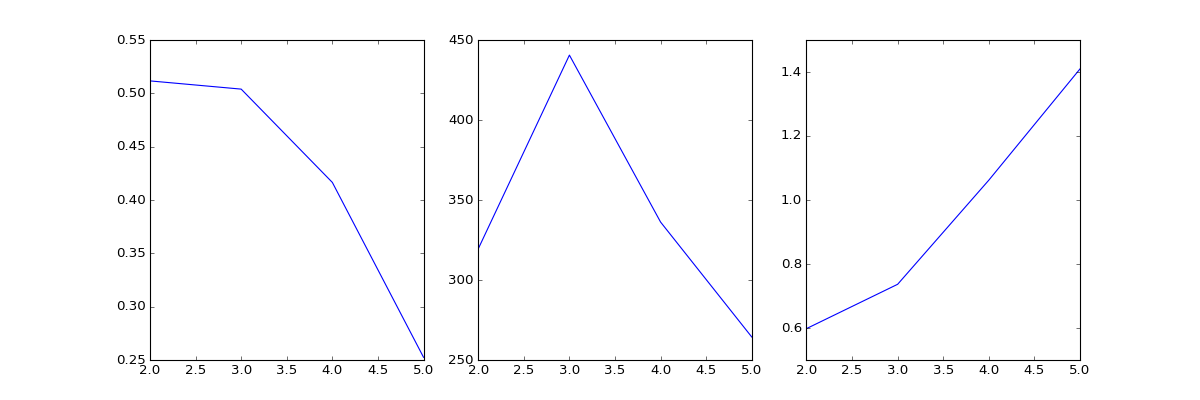And the indices have similar results

Now for non linear data

In :
from sklearn.datasets import make_moons
moons, mlabels = make_moons(n_samples=200, noise=0.1)

fig = plt.figure(figsize=(10,10))

plt.scatter(moons[:, 0], moons[:, 1], c=mlabels, s=100)
plt.show()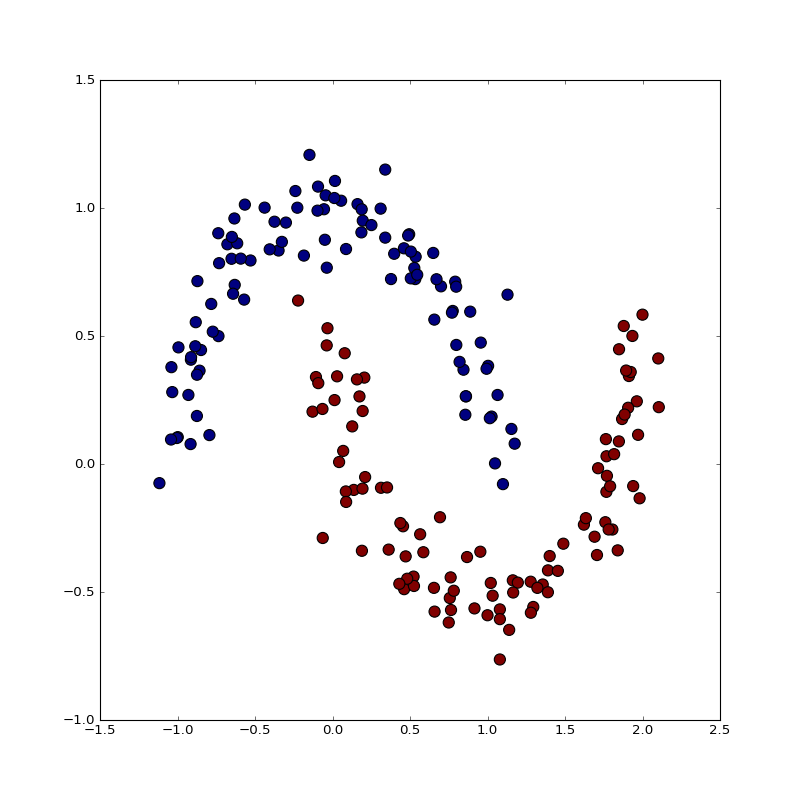Obviously the clusters obtained by K-means have little to do with the actual labels

In :
km = KMeans(n_clusters=2, n_init=10, random_state=0)
labels = km.fit_predict(moons)


0.202490375267

In :
lscores = []
nclusters = 20
for nc in range(2,nclusters+1):
km = KMeans(n_clusters=nc, n_init=10, random_state=0)
labels = km.fit_predict(moons)
lscores.append((
silhouette_score(moons, labels),
calinski_harabasz_score(moons, labels),
davies_bouldin_score(moons, labels)))

fig = plt.figure(figsize=(15,5))
plt.plot(range(2,nclusters+1), [x for x,_,_ in lscores])
plt.plot(range(2,nclusters+1), [x for _, x,_ in lscores])
plt.plot(range(2,nclusters+1), [x for _, _, x in lscores])

plt.show()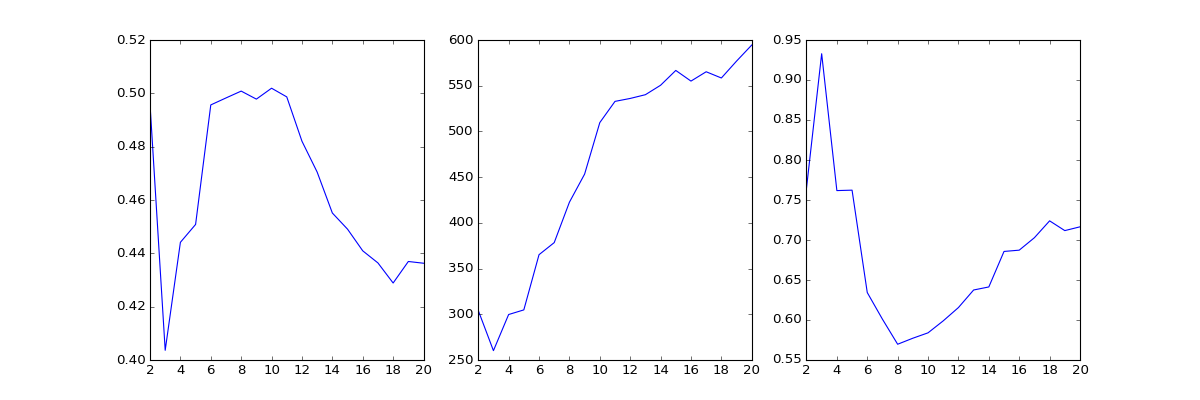Now the scores result in a high number of clusters, Silhouette and DB are close to agreeing on (maybe) 8 clusters, for CH the more the better (12-13?)

In :
lscores = []
nclusters = 20
for nc in range(2,nclusters+1):
km = KMeans(n_clusters=nc, n_init=10, random_state=0)
labels = km.fit_predict(moons)
lscores.append(scatter_matrices_scores(moons, labels, indices= ['Hartigan', 'Xu', 'ZCF']))

fig = plt.figure(figsize=(15,5))
plt.plot(range(2,nclusters+1), [x['Hartigan'] for x in lscores])
plt.plot(range(2,nclusters+1), [x['ZCF'] for x in lscores])
plt.plot(range(2,nclusters+1), [x['Xu'] for x in lscores])

plt.show()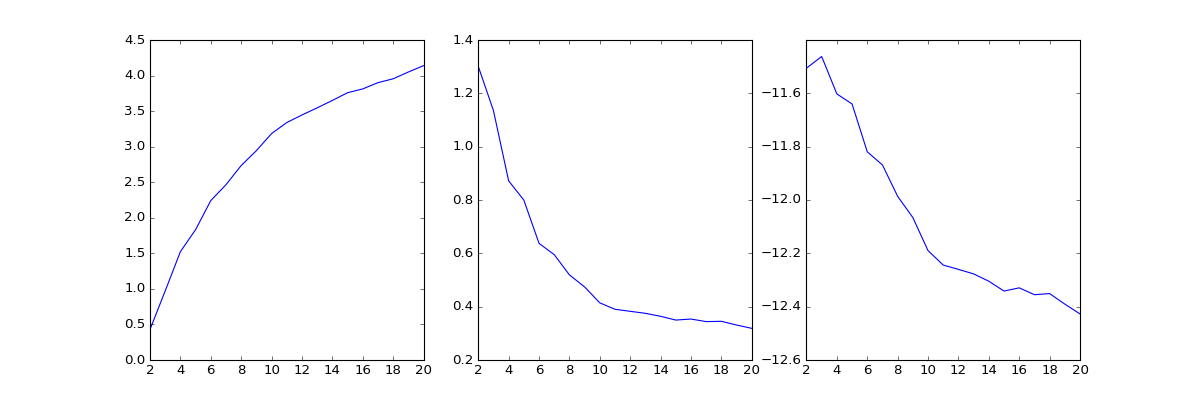The other indices also agree on the more the better

We can apply a non linear transformation to the data

In :
from sklearn.manifold import Isomap
iso = Isomap(n_components=2, n_neighbors=7)
fdata = iso.fit_transform(moons)

km = KMeans(n_clusters=2, n_init=10, random_state=0)
labels = km.fit_predict(fdata)


0.335151538367

In :
lscores = []
nclusters = 20
for nc in range(2,nclusters+1):
km = KMeans(n_clusters=nc, n_init=10, random_state=0)
labels = km.fit_predict(fdata)
lscores.append((
silhouette_score(fdata, labels),
calinski_harabasz_score(fdata, labels),
davies_bouldin_score(fdata, labels)))

fig = plt.figure(figsize=(15,5))
plt.plot(range(2,nclusters+1), [x for x,_,_ in lscores])
plt.plot(range(2,nclusters+1), [x for _, x,_ in lscores])
plt.plot(range(2,nclusters+1), [x for _, _, x in lscores])

plt.show()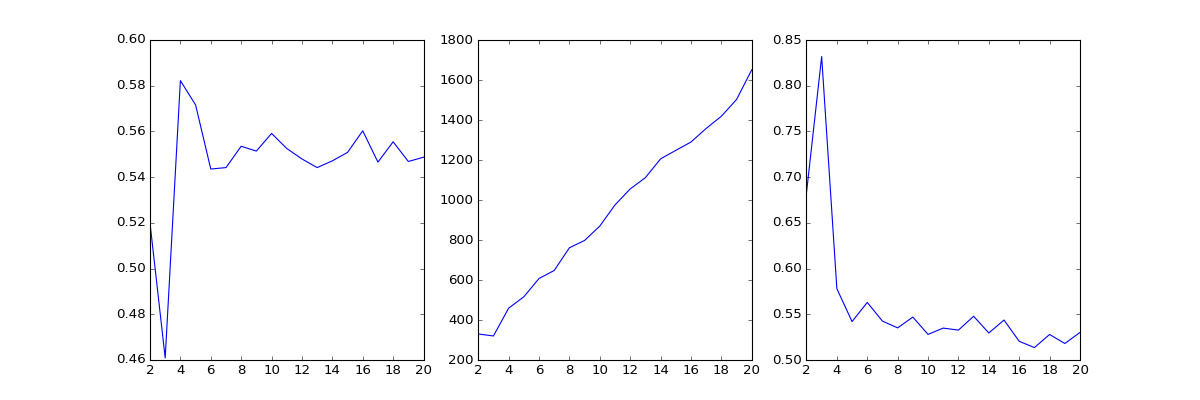But results are not much better

In :
lscores = []
nclusters = 20
for nc in range(2,nclusters+1):
km = KMeans(n_clusters=nc, n_init=10, random_state=0)
labels = km.fit_predict(fdata)
lscores.append(scatter_matrices_scores(fdata, labels, indices= ['Hartigan', 'Xu', 'ZCF']))

fig = plt.figure(figsize=(15,5))
plt.plot(range(2,nclusters+1), [x['Hartigan'] for x in lscores])
plt.plot(range(2,nclusters+1), [x['ZCF'] for x in lscores])
plt.plot(range(2,nclusters+1), [x['Xu'] for x in lscores])

plt.show()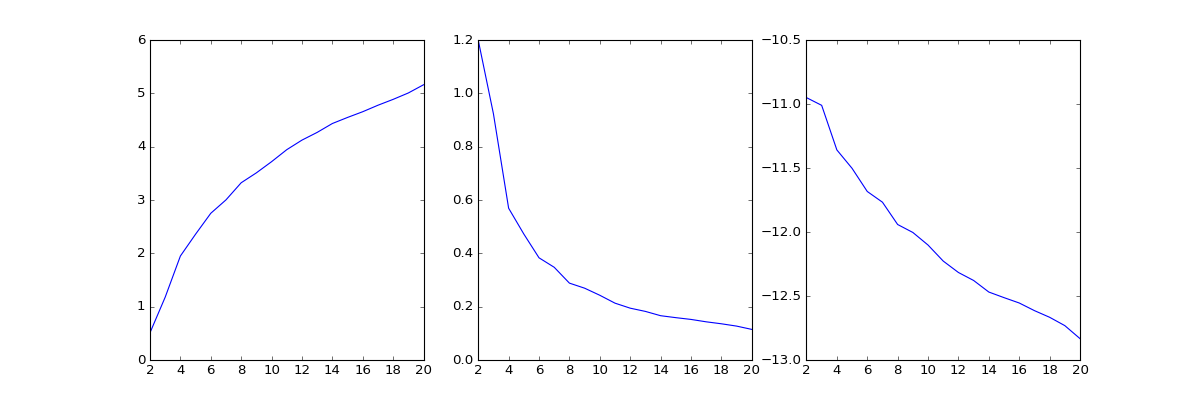For any of the indices

# External Indices¶

Another approach to cluster validation is to use external indices, this assumes that we know the labels and we compute how "correlated" are to the ones discovered.

In :
blobs, blabels = make_blobs(n_samples=500, n_features=10, centers=6, cluster_std=.6, center_box=(-3,3))

fig = plt.figure(figsize=(10,10))

ax = fig.add_subplot(111, projection='3d')
plt.scatter(blobs[:, 0], blobs[:, 1], zs=blobs[:, 2], depthshade=False, c=blabels, s=100)
plt.show()